## Rooted TreeA Tree with a special node called the Root'' or Eve.'' Denote the number of rooted trees withnodes by, then the Generating Function is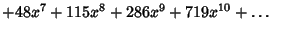(1)
(Sloane's A000081). This Power Series satisfies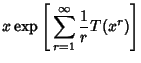(2)(3)

where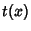is the Generating Function for unrooted Trees. A Generating Function forcan be written using a product involving the sequence itself as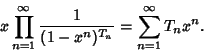(4)

The number of rooted trees can also be calculated from the Recurrence Relation(5)

with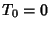and, where the second sum is over allwhich Divide(Finch).

References

Finch, S. Favorite Mathematical Constants.'' http://www.mathsoft.com/asolve/constant/otter/otter.html

Ruskey, F. Information on Rooted Trees.'' http://sue.csc.uvic.ca/~cos/inf/tree/RootedTree.html.

Sloane, N. J. A. Sequence A000081/M1180 in An On-Line Version of the Encyclopedia of Integer Sequences.'' http://www.research.att.com/~njas/sequences/eisonline.html and Sloane, N. J. A. and Plouffe, S. The Encyclopedia of Integer Sequences. San Diego: Academic Press, 1995.